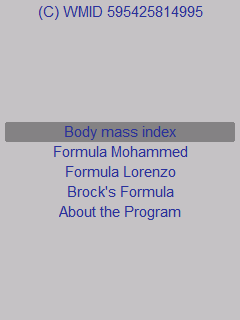### Java 2 ME Application - Ideal Weight Calculator

Java 2 ME Application - Ideal Weight Calculator, combining the most popular, widely used, and time-tested calculation methods

Broca's index

Brock's index is determined by the formula: Weight = Height - 100cm;

Body mass index

The body mass index is calculated by the formula:

BMI = weight (kg): (height (m)) 2

Lorenz formula

Ideal weight = (height (cm) - 100) - (height (cm) - 150) / 2

Formula Mohammed

Ideal weight = height * height * 0.00225

Kreff's formula

This formula takes into account age and body type.

Ideal weight = (height - 100 + (age / 10)) * 0.9 * coefficient

Odds:
Wrist less than 15 cm - coefficient 0.9
Wrist 15-17 cm - coefficient 1
Wrist more than 17 cm - a coefficient of 1.1.

Attention! This ideal weight calculator is not suitable for athletes, bodybuilders, athletes who have a high weight value, due to the large amount of muscle mass, and does not indicate the presence of obesity.In addition, it should be noted that the most accurate results of calculations are achieved with growth from 155 to 180 cm. And if growth goes beyond these limits, the error of the result greatly increases.But in any case, if you decide to seriously reduce or increase your weight, consultation with a doctor is a must.